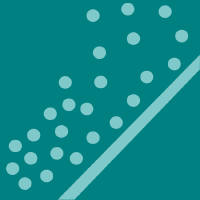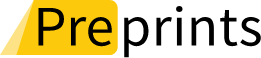## Topic Editors1. Department of Mathematics and Sciences, College of Humanities and Sciences, Prince Sultan University, Riyadh 11586, Saudi Arabia
2. Department of Mathematics, Air University, PAF Complex E-9, Islamabad 44000, PakistanDr. Syed Ahmed Pasha
Department Electrical & Computer Engineering, Air University, Islamabad 44000, PakistanDepartment of Mathematics and Sciences, College of Humanities and Sciences, Prince Sultan University, Riyadh 11586, Saudi Arabia

# Recent Advances in Statistical and Matrix Manifolds: Theory and Applications

closed (20 July 2023)
20 December 2023
Viewed by
600## Topic Information

Dear Colleagues,

Recent years have seen the uptake of information geometry to study structure of manifolds and optimization problems where probability distributions are central. The impetus has come from a number of engineering applications in signal processing, optimization, control and more recently statistical machine learning in relation to statistical inference problems and properties of statistical estimators. For example, the manifold of a positive-definite Hessian matrix arises in signal processing in the covariance matrix estimation problem of complex normal distributions and the solution of Lyapunov stability and the Riccati equations in control. Central to information geometry is the notion of a divergence measure between any two points on a manifold which endows the manifold with a Riemannian structure and a pair of dually flatness criteria. This allows generalization of the Pythagorean Theorem and the related projection theorem to manifolds. By generalizing the distance measure in the Euclidean space to a divergence, a manifold is endowed with a Riemannian structure. The information geometric approach provides a geometric interpretation for analysis which yields greater insight. Moreover, the geometric structure can be exploited to develop computationally efficient optimization algorithms. To study geometric properties of statistical and matrix manifolds and exploiting them to develop geometric optimization algorithms that will be superior to traditional methods. Homology will provide a mathematically rigorous framework to study the geometry of a general class of manifolds.

In the deterministic setting, the geometry of subgroups and submanifolds of the general linear group, that is, orthogonal group, symplectic group and special Euclidean group is of interest. In particular the left invariant metric is adopted as the Riemannian metric and the geodesic curve between any two points is defined. The exponential and logarithmic maps on these subgroups and submanifolds admit an analytical form which is convenient for computations.

For this Special Issue, we are looking for reviews or original research papers on a wide range of topics related to the information geometry and topology of manifolds and its applications in mathematics and the physical sciences.

Dr. Syed Ahmed Pasha
Dr. kamaleldin Abodayeh
Topic Editors

## Keywords

•  manifolds
•  matrix and statistical manifolds
•  information geometry
•  Riemannian manifolds
•  computational methods
•  optimizations technique
•  differentiable manifold
•  submanifold
•  curvature
•  affine connection
•  vector field
•  fiber bundle

## Participating Journals

Journal Name Impact Factor CiteScore Launched Year First Decision (median) APCAlgorithms
algorithms
2.3 3.7 2008 19.1 Days CHF 1600Axioms
axioms
2.0 2.2 2012 20 Days CHF 2400Entropy
entropy
2.7 4.7 1999 20.4 Days CHF 2600Mathematics
mathematics
2.4 3.5 2013 17.7 Days CHF 2600Symmetry
symmetry
2.7 4.9 2009 14.7 Days CHF 2400Preprints is a platform dedicated to making early versions of research outputs permanently available and citable. MDPI journals allow posting on preprint servers such as Preprints.org prior to publication. For more details about reprints, please visit https://www.preprints.org.

## Published Papers

This Topic is now open for submission.